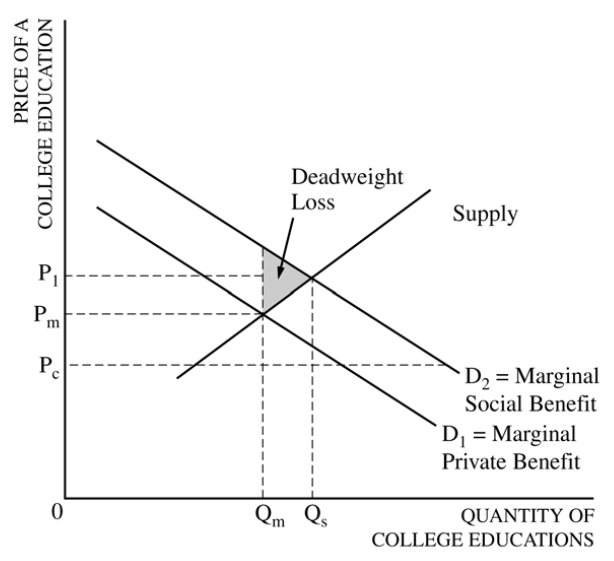# Question 1 (a)

• Graph for the market and a typical firm• Graph for a typical firm should include

• Marginal Cost

• Marginal Revenue

• Demand (Price)

• Average Total Cost

# Question 1 (d)

• Long-Run Average Total Cost

• Short-run and long-run average total cost curves differ because a firm can choose its fixed cost in the long run.

• If a firm plans on producing a high amount of output, it might make sense to have a high fixed cost

• Conversely, if a firm plans on producing a small amount of output, it might make sense to have a low fixed cost# Question 2 (a)

• Positive Social Externality# Question 2 (b)

• The price ceiling will increase the deadweight loss, because providers will decrease the quantity.

# Question 3 (c)

• the MFC curve (or the supply curve for labor) becomes horizontal at the minimum wage up to the quantity of minimum wage